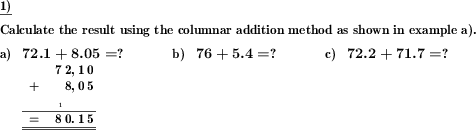Custom math worksheets at your fingertips# Details for problem "Columnar addition of decimal fractions"

Quickname: 5086

Elementary School, Primary School, Junior High School, Middle School, High School.

## Summary

Columnar addition of multiple decimal fractions.

## Example## Description

A series of positive decimal fractions has to be added in written columnar form.

The number of summands, the number of places for the summands and the number of decimal places can be selected.

The first problem may be given with the answer as an example to illustrate the way the answer is expected to be given.

Download free printable worksheets for this math problem here. The worksheet contains the problems only, the solution sheet includes the answers. Just click on the respective link.

•Worksheet 1Solution sheet with answers
•Worksheet 2Solution sheet with answers
•Worksheet 3Solution sheet with answers

If you can not see the solution sheets for download, they may be filtered out by an ad blocker that you may have installed. If this is the case, please allow ads for this page and reload the page. The solution sheets will then reappear.

• Do these sample worksheets do not really fit?
• Do you need more math worksheets, with a different level of difficulty?
• Would you like to combine different problems on a worksheet and adjust them to your needs?
• As a teacher, you can put together your own worksheets using the automatically generated math problems provided.
With a free initial credit, you can start creating your own math worksheets in a few minutes.

You can try it for free! Register here, to create custom worksheets now!

## Customization options for this problem

Parameter
Possible values
Number of problems
1, 2, 3, 4, 5, 6, 7, 8, 9, 10
Number of summands
2, 3, 4
Summand places
2, 3, 4, 5, 6, 7, 8
Summand places before point
none, one, random
Sample problem
Yes, No

## Similar problems

Remark
Description
Short form, addition of numbers in a specific range
Numbers with or without decimal places have to be added.
Short form, addition of numbers with a specific number of places
Addition of two numbers with controlled number of digits for each number.
Short or long addition of whole numbersDeutsche Version dieser Aufgabe
These informational pages with samples describe math problems that can be combined on custom math worksheets with solutions for home and K-12 school use.
Deutsche Seiten
×